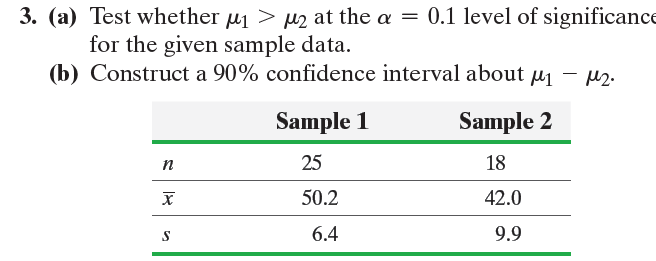Statistics: Informed Decisions Using Data - 5 Edition - Chapter 11.3 - Problem 3
Register Now

Join StudySoup

Get Full Access to Statistics: Informed Decisions Using Data - 5 Edition - Chapter 11.3 - Problem 39780134133539

# ?In Problems 1–6, assume that the populations are normally distributed. 3. (a) Test whether m1 7 m2 at the a = 0.1 level of significance for the g

Statistics: Informed Decisions Using Data | 5th Edition

Problem 3

In Problems 1–6, assume that the populations are normally distributed.

3. (a) Test whether m1 7 m2 at the a = 0.1 level of significance for the given sample data.

(b) Construct a 90% confidence interval about m1 - m2.Accepted Solution
Step-by-Step Solution:

Step 1 of 5) Assume that the populations are normally distributed. 3. (a) Test whether m1 7 m2 at the a = 0.1 level of significance for the given sample data. (b) Construct a 90% confidence interval about m1 - m2. Remember, the mean square due to error is an unbiased estimate of s2 whether the null hypothesis is true or not. However, the mean square due to treatment is an unbiased estimate of s2 only if the null hypothesis is true (that is, only if the population means from each treatment, or sample, are the same). If at least one mean for a treatment is significantly different from the others, the mean square due to treatment will substantially overestimate the value of s2 . Because the F-test statistic is the ratio of MST to MSE, ANOVA tests are always right-tailed tests. As you may have

###### Chapter 11.3, Problem 3 is Solved

Step 2 of 1

Unlock Textbook Solution